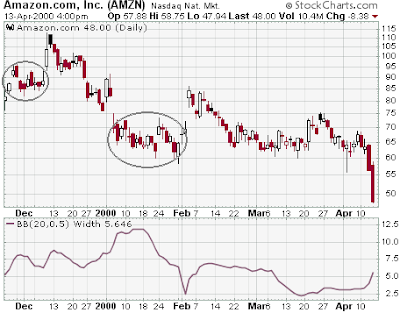Standard Deviation (Volatility)

Introduction
Standard deviation is a statistical term that provides a good indication of volatility. It measures how widely values (closing prices for instance) are dispersed from the average. Dispersion is the difference between the actual value (closing price) and the average value (mean closing price). The larger the difference between the closing prices and the average price, the higher the standard deviation will be and the higher the volatility. The closer the closing prices are to the average price, the lower the standard deviation and the lower the volatility.

Calculation

The steps for calculating a 20-period standard deviation are as follows:
1. Calculate the simple average (mean) of the closing price. i.e., Sum the last 20 closing prices and divide by 20.
2. For each period, subtract the average closing price from the actual closing price. This gives us the deviation for each period.
3. Square each period's deviation.
4. Sum the squared deviations.
5. Divide the sum of the squared deviations by the number of periods (20 in our example below).
6. The standard deviation is then equal to the square root of that number.The 20-period standard deviation for the data above is 6.787. Note that this is the "full population" version of the Standard Deviation. There is a different kind of Standard Deviation calculation that is used when you are taking a statistical sample of a population, but that version is not used in technical analysis since all of the data points are known.

Because Standard Deviation is used to determine the spread between upper and lower Bollinger Bands, the Bollinger Band Width indicator can be used as a substitute for the Standard Deviation indicator. The general shape and direction of the Bollinger Band Width indicator match that of the underlying Standard Deviation regardless of the parameters used. If you want to see the actual Standard Deviation values, set the second parameter of the Bollinger Band Width indicator to 0.5 as in this example.

Examples

The chart below shows how the standard deviation (shown via the Bollinger Band Width indicator) can change over time.After extended periods of consolidation, the standard deviation dropped. Notice that in late December the stock traded in a tight range and volatility dropped again. Later in mid-March, the stock also traded in a tight range and volatility dropped. When the stock took off in the second half of March, volatility also rose.Amazon (AMZN), which is in a similar price range as IBM, has a higher standard deviation. Until late December, the standard deviation hovered around 7.5. With the decline at the year's end, the standard deviation rose from 7 to above 12.5. Afterwards it plunged to 2.5 over the course of two weeks. Since then it leveled out around 5. This is quite a volatile stock and its options will have more premium than IBM options. The higher the volatility for a particular stock, the higher the option premiums. The lower the volatility is for a particular stock, the lower the option premiums.

Standard Deviation and SharpChartsYou can chart Standard Deviation in SharpCharts. The Parameters value is the period multiple to use. The default value is 10, which would be a 10-day Standard Deviation on a daily chart, or a 10-month Standard Deviation on a monthly chart.